# Tensor — Building block of Pytorch

Source: Deep Learning on Medium

For the sake of simplicity lets create a simple tensor with all ones to help us understand the concept better.But in real world applications the values could be anywhere between -inf to +inf.

Shape of tensor- Term used to define the dimensionalty, similar to shape in pandas DataFrames.

torch.ones : Creates tensor with given dimensions with values filled with ‘1.’

Please go through the command and the tensors printed on the command line in the left and the container representation in the right to get the sense of how tensors build from right to left.Also do read the captions below images

Since it is difficult to visualize more than 3 dimensions, we are trying to represent each dimension by a color

Tensors grow as containers in each dimension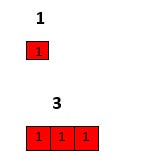We have created two tensors each of shape 1 and 3 on top and bottom respectively. When we provided 3 as dimension tensor has expanded in the red containers

Reason for this blog post- to understand where the brackets end in the tensor print in command line & it is not very obvious as dimensions grow for a beginner to get hang of it.Example below forms foundations for understanding them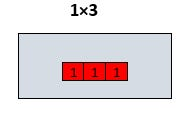We have introduced one more dimension-(1,3) . The number of items the tensor hold is still the same(3 ones) but there is one more dimension that has got added.But if we look at the way brackets are arranged the 3 dimensional tensor is now placed inside another dimension. We will understand more about it in the example below

Lets increase the value in another dimension

Lets grow the dimensions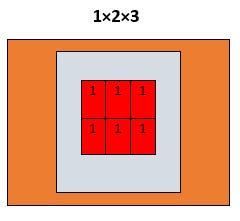Current dimensions-(1,2,3). Now the previous tensor of shape -(2,3) is now present inside another container i.e., another dimension. If we observe the way brackets ends each of them represent the way initial 3 dimensional tensor is contained in each dimension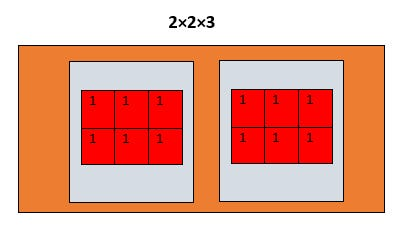Current dimension — (2,2,3). Building on the context we have till now, we are asking torch to create 2 such (2,3) tensors. In a way we are trying to grow in the container we just created. If we look at the tensor printed on cmd line on left it represents the same.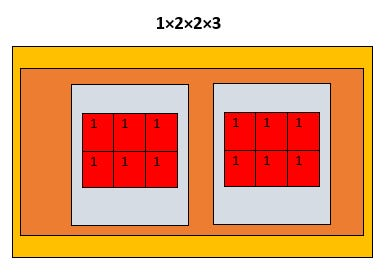Current dimensions-(1,2,2,3). We want 1 such (2,2,3). It would be more clear in example below

The last one with ones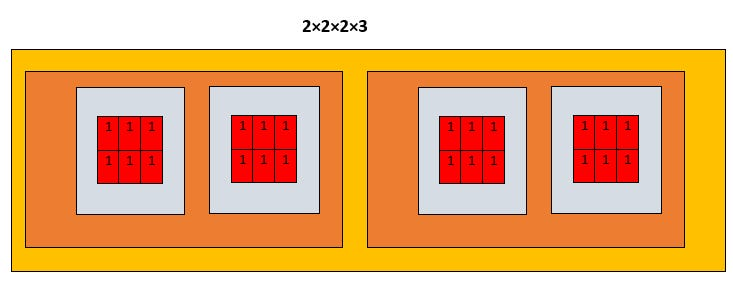Current dimension- (2,2,2,3). We are trying to create 2 tensors of dimension (2,2,3) in another dimension. Similar to the concepts discussed previously we are trying to create 2 such containers inside the new dimension we just created.

### Summary : Always read tensors from right to left.

Example:A tensor of dimension (o,p,q,r) can be interpreted as follows

q such r dimensional tensors form tensor of shape (q,r) and p such (q,r) dimensional tensors from (p,q,r) dimensional tensor and o such (p,q,r) dimensional tensors form tensor of shape — (o,p,q,r). And imagine each of them to be inside containers of other as illustrated

Question to help reinforce understanding:

What would be difference between print of the tensors of dimensions — (4,2,1,2)& (2,4,2,1). Try to do print torch.ones(given dimension) and find the answer on your command line